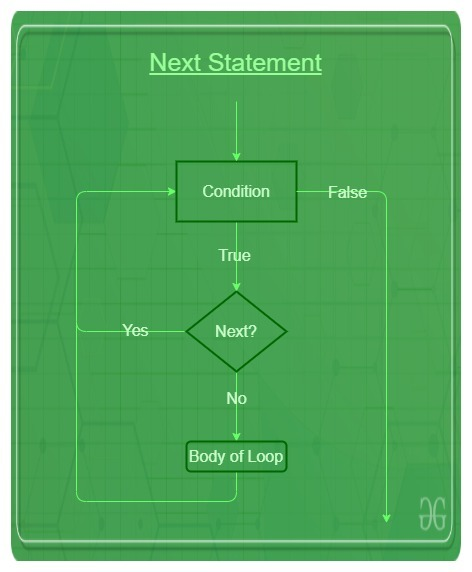Open in App
Not now

# R – Next Statement

• Last Updated : 18 Oct, 2021

Next statement in R is used to skip any remaining statements in the loop and continue the execution of the program. In other words, it is a statement that skips the current iteration without loop termination.

‘next’ is a loop control statement just like the break statement. But ‘next’ statement works opposite to that of break statement, instead of terminating the loop, it forces to execute the next iteration of the loop.

`next`

## Next Statement in R Programming Flowchart## R

 `# R program to illustrate next in for loop` `val <- 6:11` `# Loop``for ``(i ``in` `val)``{``    ``if ``(i == 8)``    ``{``        ``# test expression``        ``next` `    ``} ``    ``print``(i) ``} `

Output:

``` 6
 7
 9
 10
 11```

The next statement can be used with any other loop also like ‘while’ or ‘repeat’ loop in a similar way as it is used with for loop above.

## R

 `# R program to illustrate next in while loop` `val <- 6``i = 11``# Loop``while``(i>val)``{``    ``if ``(i == 8)``    ``{``        ``# test expression``        ``next` `    ``} ``    ``print``(i) ``    ``i = i - 1``} `

Output:

``` 11
 10
 9```

## R

 `# R program to illustrate next in repeat loop` `i = 0` `# Loop``repeat``{` `  ``if``(i == 10)   ``    ``break``    ` `  ``if``(i == 5)``  ``{   ``    ``next`      `  ``} ``    ``print``(i) ``    ``i = i + 1``} `

Output:

``` 0
 1
 2
 3
 4```

My Personal Notes arrow_drop_up